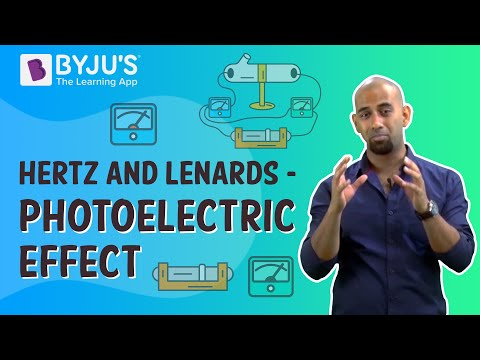# CBSE Class 12 Physics Notes Chapter 11 Dual Nature of Radiation and Matter

## What is the work function of metals?

It is referred to the minimum amount of energy required to take out one electron from the metal surface. This energy can be supplied through various sources such as a strong electric field or irradiation of light with a suitable frequency.

### What is the photoelectric effect?

It is the phenomenon in which the electrons are emitted from the metal surface when the light is illuminated in a suitable frequency. The photoelectric effect is the conversion of light to electrical energy. It also follows the law of conservation of energy and is an instantaneous process.### Factors affecting photoelectric current

Photoelectric current depends upon two factors:

• The potential difference applied within the two electrodes
• The incident light’s intensity
• The nature of the emitter material### Factors affecting stopping potential

The stopping potential is affected by:

• The incident light’s frequency
• The emitter material’s nature

The frequency of the incident light does not depend on the intensity. The stopping potential is directly proportional to the kinetic energy of the emitted electrons.

$$\begin{array}{l}eV_{0}=(1/2)mv_{max}^{2}=K_{max}\end{array}$$

We will not witness any photoelectric emission in spite of the range of intensity and this is termed as threshold frequency which is also a characteristic of metals.

### What is Quanta or Photon?

Light is composed of various discreet packs of energy which are called quanta. Einstein explained the photoelectric effect on the basis of this theory. Each photon posses an energy E=(hv) and momentum p=(h/

$$\begin{array}{l}\lambda\end{array}$$
) and it depends on the frequency (v) of light incident and never on the intensity. In metal surface, photoelectric emission occurs due to the photon absorption capability of an electron.

### Important Questions

1. 3 V is The photoelectric cut-off voltage in a certain experiment. Calculate the maximum kinetic energy of photoelectrons emitted?
2. Find the
1. maximum frequency, and
2. 50 kV electrons produce a minimum wavelength of X-rays.
1. Monochromatic light of wavelength 700 nm is produced by a helium-neon laser. The power emitted is 10 mW.
1. The momentum and energy of each photon in the light beam is?
2. Photons arrive at a target irradiated by this Beam. Find out the number of those photons (Assume the beam to have a uniform cross-section which is less than the target area), and
3. In order to have the same momentum as that of the photon, how fast does a hydrogen atom have to travel?

Related Topics:

Wave Particle Duality

Important Questions on Dual Nature of Radiation and Matter

NCERT Solutions on Dual Nature of Radiation and Matter

NCERT Exemplars on Dual Nature of Radiation and Matter

Einstein Explanation of Photoelectric Effect

Hertz And Lenard’s Observations Of The Photoelectric Effec

## Frequently asked Questions on CBSE Class 12 Physics Notes Chapter 11: Dual Nature of Radiation and Matter

### What is ‘Photoelectric effect’?

The phenomenon of emission of electrons from the surface of the metal when the light of suitable frequency falls on it is called the photoelectric effect.

### What is a ‘Magnetic field’?

The portion of space near a magnetic body or a current-carrying body in which the magnetic forces due to the body or current can be detected is called as a ‘Magnetic field’.

### What is the ‘De Broglie’s’ equation?

λ = h/mv, where λ is wavelength, h is Planck’s constant, m is the mass of a particle, moving at a velocity v. de Broglie suggested that particles can exhibit properties of waves.